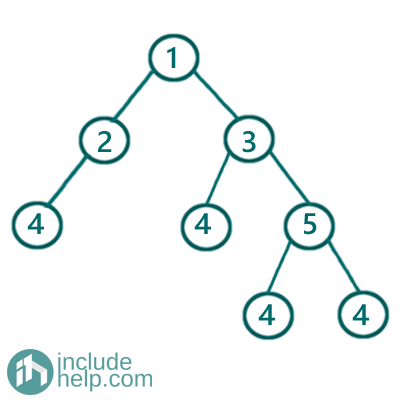# Sum of all parents having child value X in the given tree

In this article, we are going to see how to find the sum of all parents having child value X in a given tree?
Submitted by Radib Kar, on August 27, 2020

Here, we are going to see how we can use traversal techniques to solve the above problem. To do this we can use any of the traversal techniques we have learnt already. We can either use depth-first based traversal (inorder/preorder/postorder), or breadth-first based traversal (level order traversal). While traversing what we need is to keep checking whether the current node has any child with value x, if it has then we add the current node value with the cumulative sum.

In the below example,

Say X=4

We can see that the nodes 2, 3, 5 has a child with value 4 (X is 4) so the sum of all parents will be: 10Solution:

1) Using depth-first based traversals:

Here I have used inorder traversal to traverse the tree. The solution is pretty much the same as we did in Sum of all nodes in a Binary Tree. But, here we can't sum up all the nodes and we have a constraint that we can sum up nodes only having child value X. Below is the detailed implementation:

```#include <bits/stdc++.h>
using namespace std;

// tree node is defined
class TreeNode {
public:
int val;
TreeNode* left;
TreeNode* right;
TreeNode(int data)
{
val = data;
left = NULL;
right = NULL;
}
};

//depth first traversal and summing
void dfs(TreeNode* root, int x, int& sum)
{
//base cases
//1.if root is NULL return
if (!root)
return;
//2. If root is leaf return
if (!root->left && !root->right)
return;
//if any of the child has value X then add with sum
if ((root->left && root->left->val == x) || (root->right && root->right->val == x))
sum += root->val;
//traverse its left & right subtrees recursively(inorder traversal)
dfs(root->left, x, sum);
dfs(root->right, x, sum);
}

int sumofAllNodesHavingChildX(TreeNode* root, int X)
{
//variable to collect final sum
//we will pass reference so that it collects cumulatively
int sum = 0;
dfs(root, X, sum);
return sum;
}

int main()
{
//building the tree like example
TreeNode* root = new TreeNode(1);

root->left = new TreeNode(2);
root->right = new TreeNode(3);
root->left->left = new TreeNode(4);
root->right->left = new TreeNode(4);
root->right->right = new TreeNode(5);
root->right->right->left = new TreeNode(4);
root->right->right->right = new TreeNode(4);

int X;

cout << "Enter valkue of X:\n";
cin >> X;
cout << "Sum of all nodes for having child " << X << " in the given tree is: " << sumofAllNodesHavingChildX(root, X) << endl;

return 0;
}
```

Output:

```Enter valkue of X:
4
Sum of all nodes for having child 4 in the given tree is: 10
```

```#include <bits/stdc++.h>
using namespace std;

// tree node is defined
class TreeNode {
public:
int val;
TreeNode* left;
TreeNode* right;
TreeNode(int data)
{
val = data;
left = NULL;
right = NULL;
}
};

void bfs(TreeNode* root, int x, int& sum)
{
if (!root)
return;

queue<TreeNode*> q;
q.push(root);
//do a level order traversal and keep adding node
//values if the node has any child X
while (!q.empty()) {
TreeNode* temp = q.front();
q.pop();
//add current node value with sum if it has child X
if ((temp->left && temp->left->val == x) || (temp->right && temp->right->val == x))
sum += temp->val;

if (temp->left) {
q.push(temp->left);
}
if (temp->right)
q.push(temp->right);
}
}

int sumofAllNodesHavingChildX(TreeNode* root, int X)
{
//variable to collect final sum
//we will pass reference so that it collects cumulatively
int sum = 0;
bfs(root, X, sum);
return sum;
}

int main()
{
//building the tree like example
TreeNode* root = new TreeNode(1);

root->left = new TreeNode(2);
root->right = new TreeNode(3);
root->left->left = new TreeNode(4);
root->right->left = new TreeNode(4);
root->right->right = new TreeNode(5);
root->right->right->left = new TreeNode(4);
root->right->right->right = new TreeNode(4);

int X;

cout << "Enter valkue of X:\n";
cin >> X;

cout << "Sum of all nodes for having child " << X << " in the given tree is: " << sumofAllNodesHavingChildX(root, X) << endl;

return 0;
}
```

Output:

```Enter valkue of X:
4
Sum of all nodes for having child 4 in the given tree is: 10
```

What's New

Top Interview Coding Problems/Challenges!

Languages: » C » C++ » C++ STL » Java » Data Structure » C#.Net » Android » Kotlin » SQL
Web Technologies: » PHP » Python » JavaScript » CSS » Ajax » Node.js » Web programming/HTML
Solved programs: » C » C++ » DS » Java » C#
Aptitude que. & ans.: » C » C++ » Java » DBMS
Interview que. & ans.: » C » Embedded C » Java » SEO » HR
CS Subjects: » CS Basics » O.S. » Networks » DBMS » Embedded Systems » Cloud Computing
» Machine learning » CS Organizations » Linux » DOS
More: » Articles » Puzzles » News/Updates